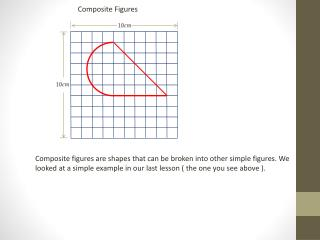DownloadDownload PresentationComposite Figures

# Composite Figures

Télécharger la présentation## Composite Figures

- - - - - - - - - - - - - - - - - - - - - - - - - - - E N D - - - - - - - - - - - - - - - - - - - - - - - - - - -
##### Presentation Transcript

1. Composite Figures Composite figures are shapes that can be broken into other simple figures. We looked at a simple example in our last lesson ( the one you see above ).

2. Composite Figures Composite figures are shapes that can be broken into other simple figures. We looked at a simple example in our last lesson ( the one you see above ). This figure was a composite of a triangle and ½ of a circle.

3. Composite Figures This lesson will look at finding the perimeter and area of more complex composite shapes …

4. Composite Figures Find the perimeter and area of the figure. 10 inches 6 inches 18 inches

5. Composite Figures Find the perimeter and area of the figure. For perimeter, so far we know we have an 18 inch side and a 6 inch side. 10 inches 6 inches 18 inches

6. Composite Figures Find the perimeter and area of the figure. For perimeter, so far we know we have an 18 inch side and a 6 inch side. We need to find the diagonal… 10 inches 6 inches 18 inches

7. Composite Figures Find the perimeter and area of the figure. For perimeter, so far we know we have an 18 inch side and a 6 inch side. We need to find the diagonal… and the curved distance… 10 inches 6 inches 18 inches

8. Composite Figures Find the perimeter and area of the figure. For perimeter, so far we know we have an 18 inch side and a 6 inch side. We need to find the diagonal… and the curved distance… 10 inches 6 inches 18 inches The diagonal is the hypotenuse of a triangle with a base of 8 inches ( 18 – 10 ), and a height of 6 inches…

9. Composite Figures Find the perimeter and area of the figure. For perimeter, so far we know we have an 18 inch side and a 6 inch side. We need to find the diagonal… and the curved distance… 10 inches 6 inches 18 inches

10. Composite Figures Find the perimeter and area of the figure. For perimeter, so far we know we have an 18 inch side and a 6 inch side. We need to find the diagonal… and the curved distance… 10 inches 6 inches 18 inches The curved distance is the circumference of ½ of a circle with diameter = 10 inches

11. Composite Figures Find the perimeter and area of the figure. For perimeter, so far we know we have an 18 inch side and a 6 inch side. We need to find the diagonal… and the curved distance… 10 inches 6 inches 18 inches

12. Composite Figures Find the perimeter and area of the figure. For perimeter, so far we know we have an 18 inch side and a 6 inch side. We need to find the diagonal… and the curved distance… 10 inches 6 inches 18 inches

13. Composite Figures Find the perimeter and area of the figure. For the area of the figure, we will need to find the area of the rectangle, triangle, and ½ circle, then add them together… 10 inches 6 inches 18 inches

14. Composite Figures Find the perimeter and area of the figure. For the area of the figure, we will need to find the area of the rectangle, triangle, and ½ circle, then add them together… 10 inches 6 inches 18 inches

15. Composite Figures Find the perimeter and area of the figure. For the area of the figure, we will need to find the area of the rectangle, triangle, and ½ circle, then add them together… 10 inches 6 inches 18 inches

16. Composite Figures Find the perimeter and area of the figure. For the area of the figure, we will need to find the area of the rectangle, triangle, and ½ circle, then add them together… 10 inches 6 inches 18 inches

17. Composite Figures Find the perimeter and area of the figure. For the area of the figure, we will need to find the area of the rectangle, triangle, and ½ circle, then add them together… 10 inches 6 inches 18 inches